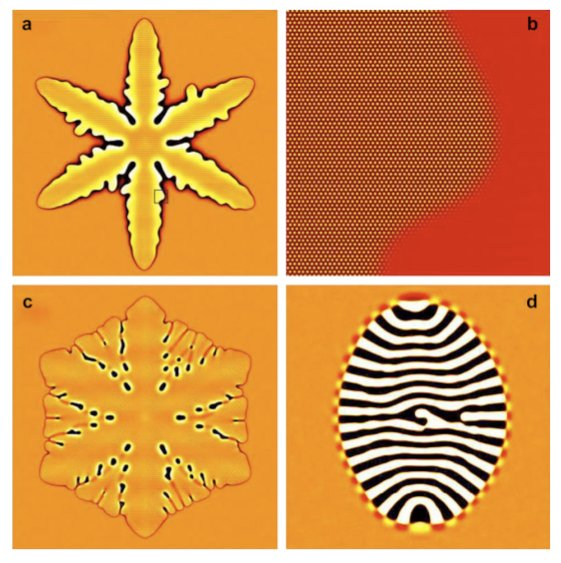## Advanced operator-splitting-based semi-implicit spectral method to solve the binary phase-field crystal equation with variable coefficientsGyörgy Tegze1, Gurvinder Bansel2, Gyula Tóth3, Tamás Pusztai1, Zhongyun Fan2, László Gránásy1,2

1Institute for Solid State Physics and Optics, Wigner Research Centre for Physics, P.O. Box 49, Budapest H-1525, Hungary
2BCAST, Brunel University, Uxbridge, Middlesex, UB8 3PH, United Kingdom
3Department of Mathematical Sciences, Loughborough University, Loughborough, Leicestershire, LE11 3TU, U.K.

We present an efficient method to solve numerically the equations of dissipative dynamics of the binary phase-field crystal model proposed by Elder et al. [K.R. Elder, M. Katakowski, M. Haataja, M. Grant, Phys. Rev. B 75, 064107 (2007)] characterized by variable coefficients. Using the operator splitting method, the problem has been decomposed into sub-problems that can be solved more efficiently. A combination of non-trivial splitting with spectral semi-implicit solution leads to sets of algebraic equations of diagonal matrix form. Extensive testing of the method has been carried out to find the optimum balance among errors associated with time integration, spatial discretization, and splitting. We show that our method speeds up the computations by orders of magnitude relative to the conventional explicit finite difference scheme, while the costs of the pointwise implicit solution per timestep remains low. Also we show that due to its numerical dissipation, finite differencing can not compete with spectral differencing in terms of accuracy. In addition, we demonstrate that our method can efficiently be parallelized for distributed memory systems, where an excellent scalability with the number of CPUs is observed.

Topics: Phase field crystal